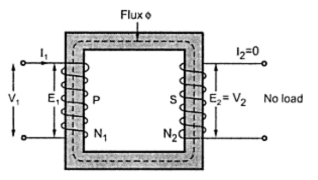Ideal Transformer on No Load

Consider an ideal transformer on no load as shown in the Fig. 3. The supply voltage is and as it is V1 an no load the secondary current I2 = 0.
The primary draws a current I1 which is just necessary to produce flux in the core. As it magnetising the core, it is called magnetising current denoted as Im. As the transformer is ideal, the winding resistance is zero and it is purely inductive in nature. The magnetising current is Im is very small and lags V1 by 30o as the winding is purely inductive. This Im produces an alternating flux Φ which is in phase with Im.Fig.  1  Ideal transformer on no load

The flux links with both the winding producing the induced e.m.f.s E1 and E2 , in the primary and secondary windings respectively. According to Lenz's law, the induced e.m.f. opposes the cause producing it which is supply voltage V1. Hence E1 is in antiphase with V1 but equal in magnitude. The induced E2 also opposes V1 hence in antiphase with V1 but its magnitude depends on N2. Thus E1 and E2 are in phase.
The phasor diagram for the ideal transformer on no load is shown in the Fig. .2.Fig.  2  Phasor diagram for ideal transformer on no load
It can be seen that flux Φ is reference. Im produces Φ hence in phase with Φ. V1 leads Im by 90o as winding is purely inductive so current has to lag voltage by 90o.
E1 and E2 are in phase and both opposing supply voltage .
The power input to the transformer is V1 I1 cos (V1 ^ I1 ) i.e. V1 Im cos(90o) i.e. zero. This is because on no load output power is zero and for ideal transformer there are no losses hence input power is also zero. Ideal no load p.f. of transformer is zero lagging.

1.